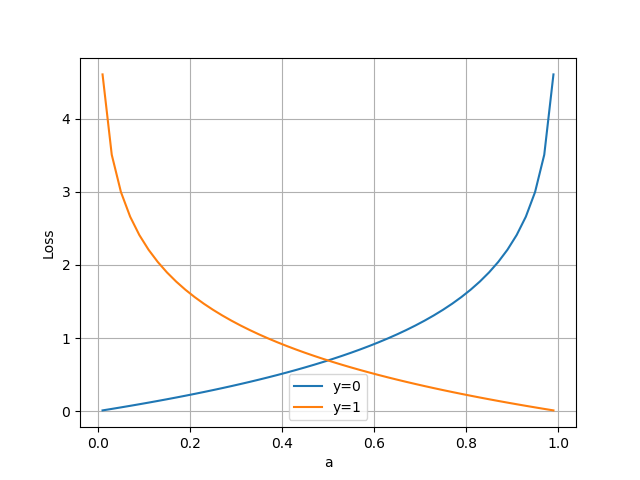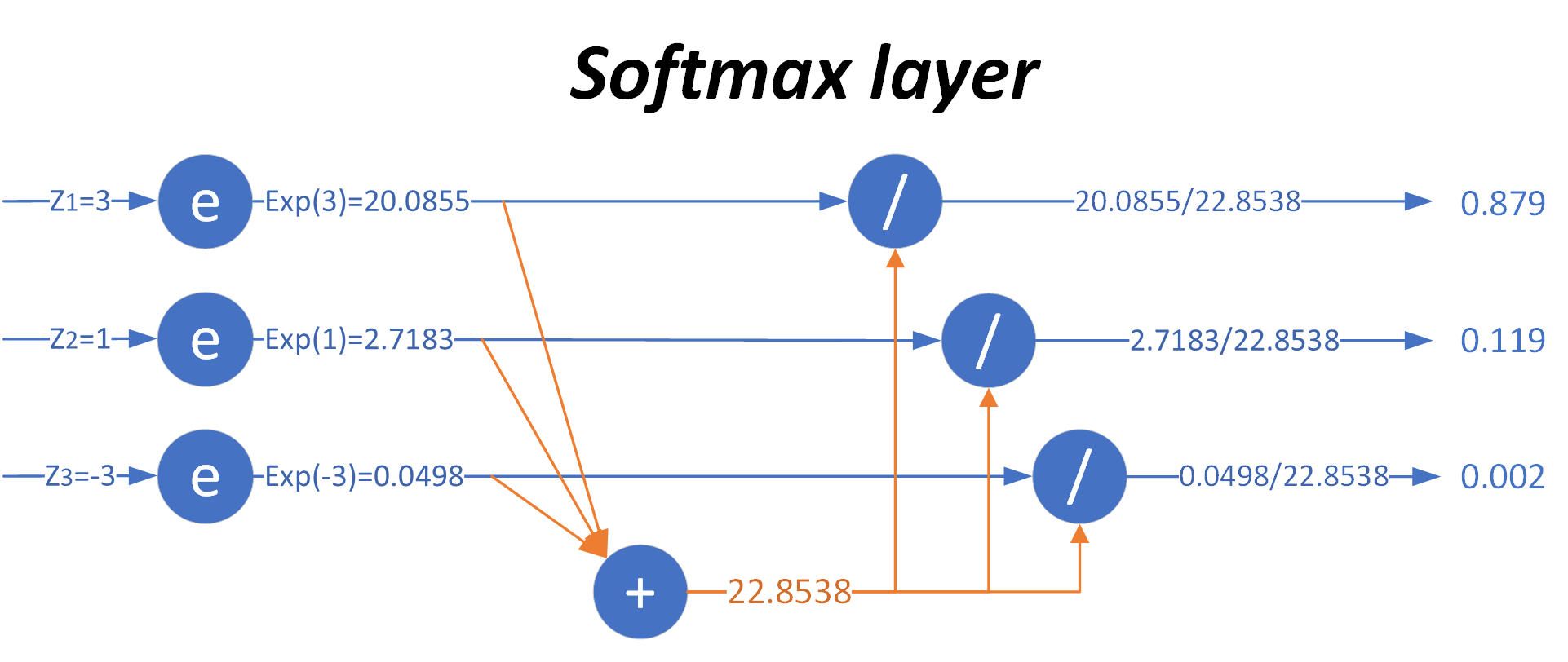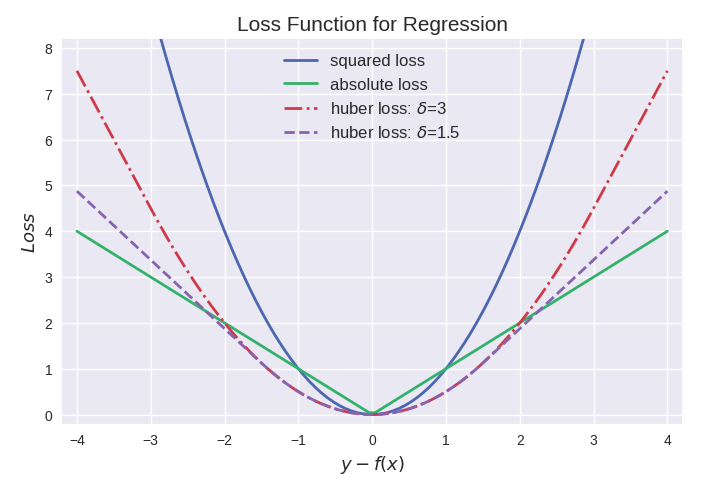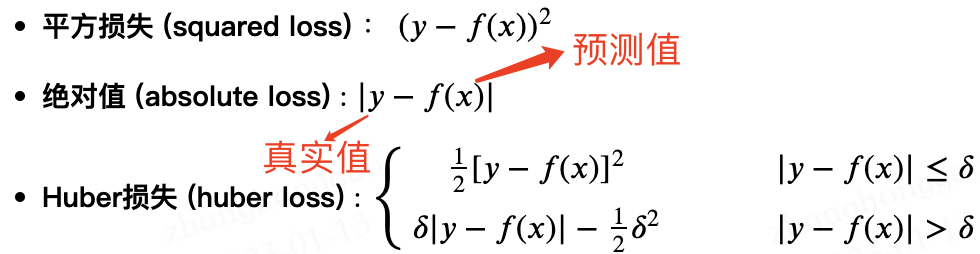# 深度學習基礎-損失函式詳解

## 二，交叉熵函式-分類損失

### 2.1，交叉熵（Cross-Entropy）的由來

1，資訊量

《深度學習》（花書）中稱為自資訊(self-information) 。 在本文中，我們總是用 $\text{log}$ 來表示自然對數，其底數為 $e$。

$$I(x_j) = -\log (p(x_j))$$

• $x_j$：表示一個事件
• $p(x_j)$：表示事件 $x_j$ 發生的概率
• $I(x_j)$：資訊量，$x_j$ 越不可能發生時，它一旦發生後的資訊量就越大

2，熵

$$H(p) = - \sum_j^n p(x_j) \log (p(x_j))$$

\begin{aligned} H(p)&=-[p(x_1) \ln p(x_1) + p(x_2) \ln p(x_2) + p(x_3) \ln p(x_3)] \\ &=0.7 \times 0.36 + 0.2 \times 1.61 + 0.1 \times 2.30 \\ &=0.804 \end{aligned}

3，相對熵(KL散度)

KL散度的計算公式：

$$D_{KL}(p||q)=\sum_{j=1}^m p(x_j) \log {p(x_j) \over q(x_j)}$$

$m$ 為事件的所有可能性（分類任務中對應類別數目）。$D$ 的值越小，表示 $q$ 分佈和 $p$ 分佈越接近

4，交叉熵

\begin{aligned} D_{KL}(p||q)&=\sum_{j=1}^m p(x_j) \log {p(x_j)} - \sum_{j=1}^m p(x_j) \log q(x_j) \\ &=- H(p(x)) + H(p,q) \end{aligned}

$$H(p,q) =- \sum_{j=1}^m p(x_j) \log q(x_j)$$

$$loss = \sum_{j = 1}^{m}y_{j}\text{log}(a_{j})$$

$$J = -\frac{1}{n}\sum_{i=1}^n \sum_{j=1}^{m} y_{ij} \log a_{ij}$$

$$loss =-[y \log a + (1-y) \log (1-a)]$$

$$J= -\frac{1}{n} \sum_{i=1}^n [y_i \log a_i + (1-y_i) \log (1-a_i)]$$

#### 2.1.1，熵、相對熵以及交叉熵總結

\begin{aligned} H(p) &= -\sum_{j}p(x_j) \log p(x_j) \ D_{KL}(p \parallel q) &= \sum_{j}p(x_j)\log \frac{p(x_j)}{q(x_j)} = \sum_j (p(x_j)\log p(x_j) - p(x_j) \log q(x_j)) \ H(p,q) &= -\sum_j p(x_j)\log q(x_j) \ \end{aligned}

### 2.2，二分類問題的交叉熵### 2.3，多分類問題的交叉熵

|預測值|標籤值|是否正確| |-----|-----|-------| |0.3 0.3 0.4|0 0 1（豬）|正確| |0.3 0.4 0.4|0 1 0（狗）|正確| |0.1 0.2 0.7|1 0 0（貓）|錯誤|

|預測值|標籤值|是否正確| |-----|-----|-------| |0.1 0.2 0.7|0 0 1（豬）|正確| |0.1 0.7 0.2|0 1 0（狗）|正確| |0.3 0.4 0.4|1 0 0（貓）|錯誤|

\begin{aligned} \text{sample}\ 1\ \text{loss} = -(0\times log(0.3) + 0\times log(0.3) + 1\times log(0.4) = 0.91 \ \text{sample}\ 1\ \text{loss} = -(0\times log(0.3) + 1\times log(0.4) + 0\times log(0.4) = 0.91 \ \text{sample}\ 1\ \text{loss} = -(1\times log(0.1) + 0\times log(0.2) + 0\times log(0.7) = 2.30 \end{aligned}

$$L = \frac{0.91 + 0.91 + 2.3}{3} = 1.37$$

\begin{aligned} \text{sample}\ 1\ \text{loss} = -(0\times log(0.1) + 0\times log(0.2) + 1\times log(0.7) = 0.35 \ \text{sample}\ 1\ \text{loss} = -(0\times log(0.1) + 1\times log(0.7) + 0\times log(0.2) = 0.35 \ \text{sample}\ 1\ \text{loss} = -(1\times log(0.3) + 0\times log(0.4) + 0\times log(0.4) = 1.20 \end{aligned}

$$L = \frac{0.35 + 0.35 + 1.2}{3} = 0.63$$

### 2.4，PyTorch 中的 Cross Entropy

PyTorch 中常用的交叉熵損失函式為 torch.nn.CrossEntropyLoss

python class torch.nn.CrossEntropyLoss(weight=None, size_average=None, ignore_index=-100, reduce=None, reduction='elementwise_mean')

1，函式功能:

2，引數解釋:

• weight(Tensor)- 為每個類別的 loss 設定權值，常用於類別不均衡問題。weight 必須是 float 型別的 tensor，其長度要於類別 C 一致，即每一個類別都要設定有 weight。
• size_average(bool)- 當 reduce=True 時有效。為 True 時，返回的 loss 為平均值;為 False 時，返回的各樣本的 loss 之和。
• reduce(bool)- 返回值是否為標量，預設為 True。
• ignore_index(int)- 忽略某一類別，不計算其 loss，其 loss 會為 0，並且，在採用 size_average 時，不會計算那一類的 loss，除的時候的分母也不會統計那一類的樣本。

#### 2.4.1，Softmax 多分類函式

Softmax 從字面上來說，可以分成 soft 和 max 兩個部分。max 故名思議就是最大值的意思。Softmax 的核心在於 soft，而 soft 有軟的含義，與之相對的是 hard 硬，即 herdmax。下面分佈演示將模型輸出值取 max 值引入 Softmax 的對比情況。

1. 分類結果是 $[1,0,0]$，只保留非 0 即 1 的資訊，即非黑即白，沒有各元素之間相差多少的資訊，可以理解是“Hard Max”；
2. max 操作本身不可導，無法用在反向傳播中。

Softmax 加了個"soft"來模擬 max 的行為，但同時又保留了相對大小的資訊。

$$a_j = \text{Softmax}(z_j) = \frac{e^{z_j}}{\sum\limits_{i=1}^m e^{z_i}}=\frac{e^{z_j}}{e^{z_1}+e^{z_2}+\dots+e^{z_m}}$$

• $z_j$ 是對第 $j$ 項的分類原始值，即矩陣運算的結果
• $z_i$ 是參與分類計算的每個類別的原始值
• $m$ 是總分類數
• $a_j$ 是對第 $j$ 項的計算結果|輸入原始值|MAX計算|Softmax計算| |--------|-------|----------| |$[3, 1, -3]$|$[1, 0, 0]$|$[0.879, 0.119, 0.002]$|

1. 三個類別的概率相加為 1
2. 每個類別的概率都大於 0

python

# example of the argmax of a list of numbers

from numpy import argmax from numpy import exp

# define data

data = [3, 1, -3]

def hardmax(data): """# calculate the argmax of the list""" result = argmax(data) return result

def softmax(vector): """# calculate the softmax of a vector""" e = exp(vector) return e / e.sum()

hardmax_result = hardmax(data)

# 執行該示例返回列表索引值“0”，該值指向包含列表“3”中最大值的陣列索引 。

print(hardmax(data)) # 0

# convert list of numbers to a list of probabilities

softmax_result = softmax(data) print(softmax_result) # report the probabilities print(sum(softmax_result)) # report the sum of the probabilitie 

0

[0.87887824 0.11894324 0.00217852]

1.0

Pytorch 中的 Softmax 函式定義如下:

python def softmax(x): return torch.exp(x)/torch.sum(torch.exp(x), dim=1).view(-1,1)

dim=1 用於 torch.sum() 對所有列的每一行求和，.view(-1,1) 用於防止廣播。

## 三，迴歸損失

$$l_{t}^{i} = y_{t}^{i} - \hat{y}_{t}^{i}$$

### 3.2，MSE 損失

$$\text{MSE loss} = \frac{1}{n}\sum_{i=1}^{N}\sum_{t=1}^{M} (y_{t}^{i} - \hat{y}_{t}^{i})^2$$

### 3.3，Huber 損失

MAE 和 MSE 損失之間的比較產生以下結果：

1. MAE 損失比 MSE 損失更穩健。仔細檢視公式，可以觀察到如果預測值和實際值之間的差異很大，與 MAE 相比，MSE 損失會放大效果。 由於 MSE 會屈服於異常值，因此 MAE 損失函式是更穩健的損失函式。

2. MAE 損失不如 MSE 損失穩定。由於 MAE 損失處理的是距離差異，因此一個小的水平變化都可能導致迴歸線波動很大。在多次迭代中發生的影響將導致迭代之間的斜率發生顯著變化。總結就是，MSE 可以確保迴歸線輕微移動以對資料點進行小幅調整。

3. MAE 損失更新的梯度始終相同。即使對於很小的損失值，梯度也很大。這樣不利於模型的學習。為了解決這個缺陷，我們可以使用變化的學習率，在損失接近最小值時降低學習率。
4. MSE 損失的梯度隨損失增大而增大，而損失趨於0時則會減小。其使用固定的學習率也可以有效收斂。

Huber Loss 結合了 MAE 的穩健性和 MSE 的穩定性，本質上是 MAE 和 MSE 損失中最好的。對於大誤差，它是線性的，對於小誤差，它本質上是二次的

Huber Loss 的特徵在於引數 $\delta$。當 $|y − \hat{y}|$ 小於一個事先指定的值 $\delta$ 時，變為平方損失，大於 $\delta$ 時，則變成類似於絕對值損失，因此其是比較robust 的損失函式。其定義如下:

$$\text{Huber loss} = \left \lbrace \begin{matrix} \frac12[y_{t}^{i} - \hat{y}{t}^{i}]^2 & |y{t}^{i} - \hat{y}{t}^{i}| \leq \delta \ \delta|y{t}^{i} - \hat{y}{t}^{i}| - \frac12\delta^2 & |y{t}^{i} - \hat{y}_{t}^{i})| > \delta \end{matrix}\right.$$### 3.4，程式碼實現

python

# pred: Array of predictions

def mse(true, pred): return np.sum((true - pred)**2)

def mae(true, pred): return np.sum(np.abs(true - pred))

def huber(true, pred, delta): loss = np.where(np.abs(true-pred) < delta , 0.5((true-pred)2),deltanp.abs(true - pred) - 0.5(delta*2))

return np.sum(loss)


# also available in sklearn

from sklearn.metrics import mean_squared_error from sklearn.metrics import mean_absolute_error 

## 參考資料

1. 《動手學深度學習-22.11. Information Theory》
2. 損失函式｜交叉熵損失函式
3. AI-EDU: 交叉熵損失函式
4. 常見迴歸和分類損失函式比較
5. 《PyTorch_tutorial_0.0.5_餘霆嵩》
6. https://pytorch.org/docs/stable/generated/torch.nn.CrossEntropyLoss.html
7. 一文詳解Softmax函式
8. AI-EDU: 多分類函式
「其他文章」